# Control Flow Statements in Python

## Control Flow Statements in Python

In this article, I am going to discuss Control Flow Statements in Python with Examples. Please read our previous article where we discussed Input and Output in Python with examples. At the end of this article, you will understand the following pointers in detail.

1. Why should we learn about flow control?
2. What is Flow control in Python?
3. Sequential flow
4. Conditional flow
5. Looping flow
6. Sequential statements
7. Conditional or Decision-making statements
8. If statement
9. If else statement
10. If elif else statement
11. Multiple Programs to understand all the above concepts
##### Why should we learn about flow control?

In programming languages, flow control means the order in which the statements or instructions, that we write, get executed. In order to understand a program, we should be aware of what statements are being executed and in which order. So, understanding the flow of the program is very important. There are, generally, three ways in which the statements will be executed. They are,

1. Sequential
2. Conditional
3. Looping
##### Flow control graphs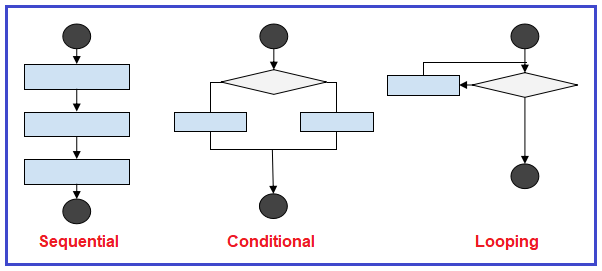Sequential: In this type of execution flow, the statements are executed one after the other sequentially. By using sequential statements, we can develop simple programs

Conditional: Statements are executed based on the condition. As shown above in the flow graph, if the condition is true then one set of statements are executed, and if false then the other set. Conditional statements are used much in complex programs.

Looping(iteration): A set of statements are executed repeatedly until the condition becomes false. Once the condition becomes false then the execution comes out of that loop.

##### SEQUENTIAL STATEMENTS IN PYTHON:

As stated above the sequential statements are executed line by line.

###### Example: Sequential statements (demo1.py)
```print("one")
print("two")
print("three")```
###### Output:##### CONDITIONAL STATEMENTS IN PYTHON

Conditional statements are also called decision-making statements. Let’s discuss some conditions making statements in detail. There are three types of conditional statements in python. They are as follows:

1. if statement
2. if-else statement
3. if elif else
##### if statement in python: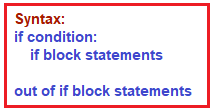The if statement contains an expression/condition. As per the syntax colon (:) is mandatory otherwise it throws a syntax error. The condition gives the result as a bool type, either True or False. If the condition result is True, then if block statements will be executed. If the condition result is False, then if block statements won‟t execute.

###### Example: if statement (demo2.py)
```num = 1
print("num==1 condition gives: ", (num==1))
if num == 1:
print("if block statements executed")```
###### Output: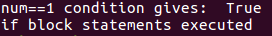##### Example: if statement (demo3.py)
```num = 1
print("num==2 condition gives: ", (num==1))
if num == 2:
print("if block statements executed")
print("out of if block statements")```
###### Output: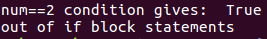The statements that should be executed if the condition is true, should have one level indentation with respect to the if clause. In the demo2.py the condition ‘num == 1’ is evaluated to True, hence the print function inside the if the condition is executed. In the demo3.py the condition ‘num == 2’ is evaluated to False, hence the print function outside the if the condition is executed.

##### if-else statement in python: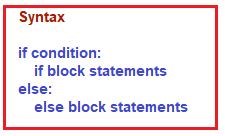The if statement contains an expression/condition. As per the syntax colon (:) is mandatory after the condition and after the else otherwise it throws a syntax error. The condition gives the result as bool type, which means either True or False. If the condition result is True, then if block statements will be executed. If the condition result is False, then else block statements will be executed.

###### Example: if-else statement (demo4.py)
```num = 1
print("num==1 condition gives: ", (num==1))
if num == 1:
print("if block statements executed")
else:
print("else block statements executed")```
###### Output: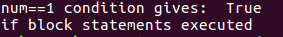##### Example: if-else statement (demo5.py)
```num = 1
print("num==2 condition gives: ", (num==1))
if num == 2:
print("if block statements executed")
else:
print("else block statements executed")```
###### Output: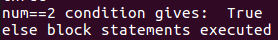The if block statements and else block statements should have one level indentation. (highlighted with blue color). In the demo4.py the condition ‘num == 1’ is evaluated to True, hence the print function inside the if the condition is executed. In the demo5.py the condition ‘num == 2’ is evaluated to False, hence the print function inside the else is executed.

##### if elif else statement in python: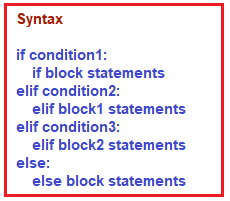The if statement contains an expression/condition. As per the syntax colon (:) is mandatory after each condition and after the else otherwise it throws a syntax error. The condition gives the result as bool type, which means either True or False. The conditions are evaluated in the order written. If one condition becomes True then the set of statements associated with that particular condition are executed and the execution comes out without checking other conditions.

###### Example: if elif statement (demo6.py)
```print("Please enter the values from 0 to 4")
x=int(input("Enter a number: "))

if x==0:
print("You entered:", x)
elif x==1:
print("You entered:", x)
elif x==2:
print("You entered:", x)
elif x==3:
print("You entered:", x)
elif x==4:
print("You entered:", x)
else:
print("Beyond the range than specified")```
###### Output1: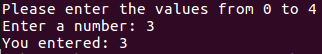###### Output2: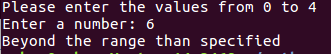##### Example: Checking number is positive or negative using if-else statement (demo7.py)
```x=int(input("Enter a number: "))
if x<0:
print("The number is negative number")

else:
print("The number is positive number")```
###### Output1: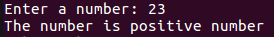###### Output2: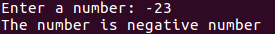###### Example: Validating the name entered with the username we have (demo8.py)
```user_name = 'rahul'
x=input("Enter a name: ")
if user_name == x:
print("The name is valid")
else:
print("The name is invalid")```
###### Output1: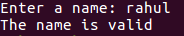###### Output2: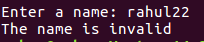###### Example: Finding the biggest number among entered two numbers (demo9.py)
```x=int(input("Enter First Number: "))
y=int(input("Enter Second Number: "))
if x>y :
print("Biggest Number is: ", x)
else :
print("Biggest Number is: ", y)```
###### Output: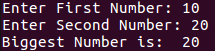###### Example: Finding the biggest number among entered three numbers (demo10.py)
```x=int(input("Enter First Number: "))
y=int(input("Enter Second Number: "))
z=int(input("Enter Third Number: "))
if x>y  and x>z:
print("Biggest Number is: ", x)
elif y>z :
print("Biggest Number is: ", y)
else:
print("Biggest Number is: ", z)```
###### Output: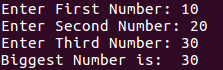1.